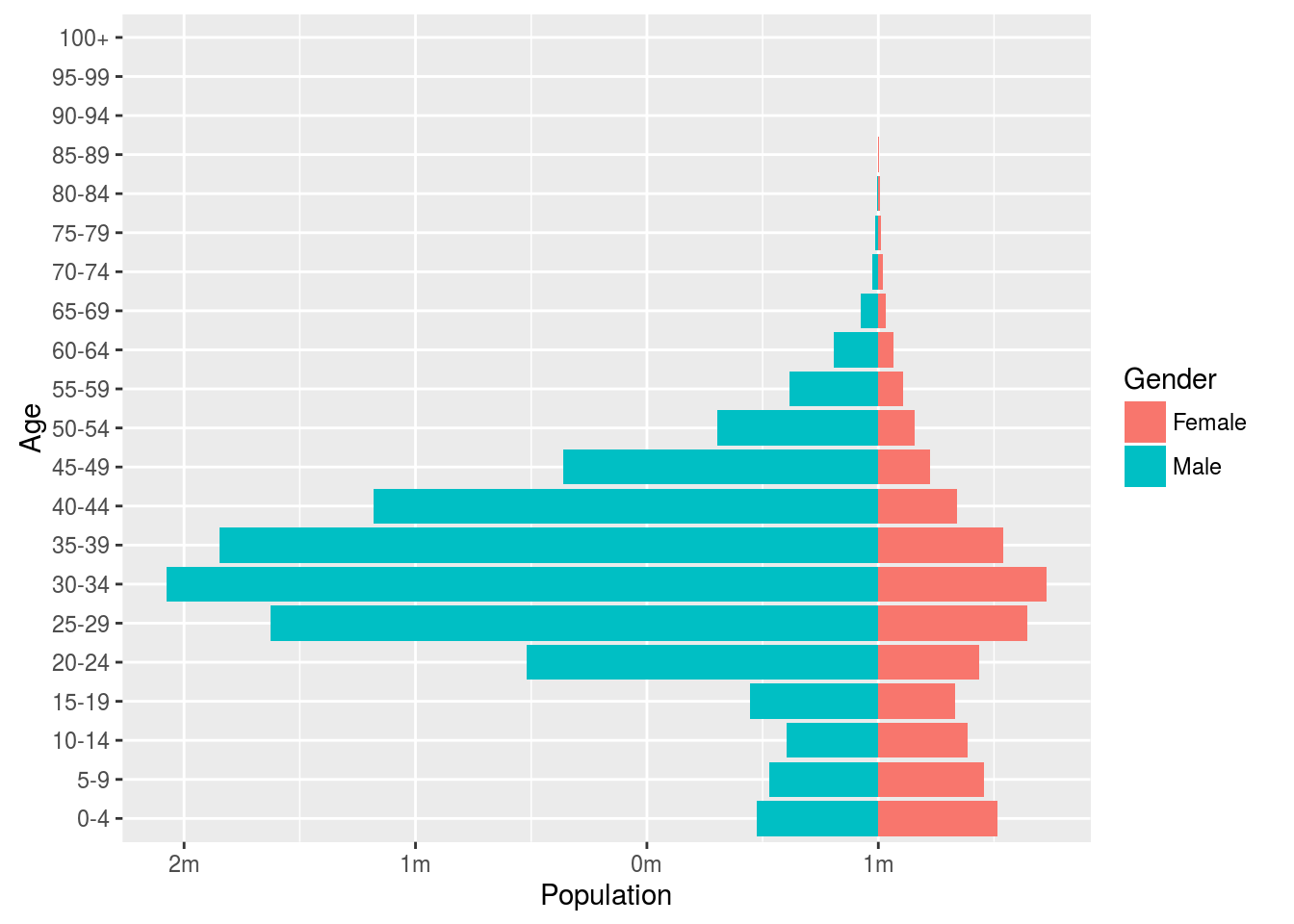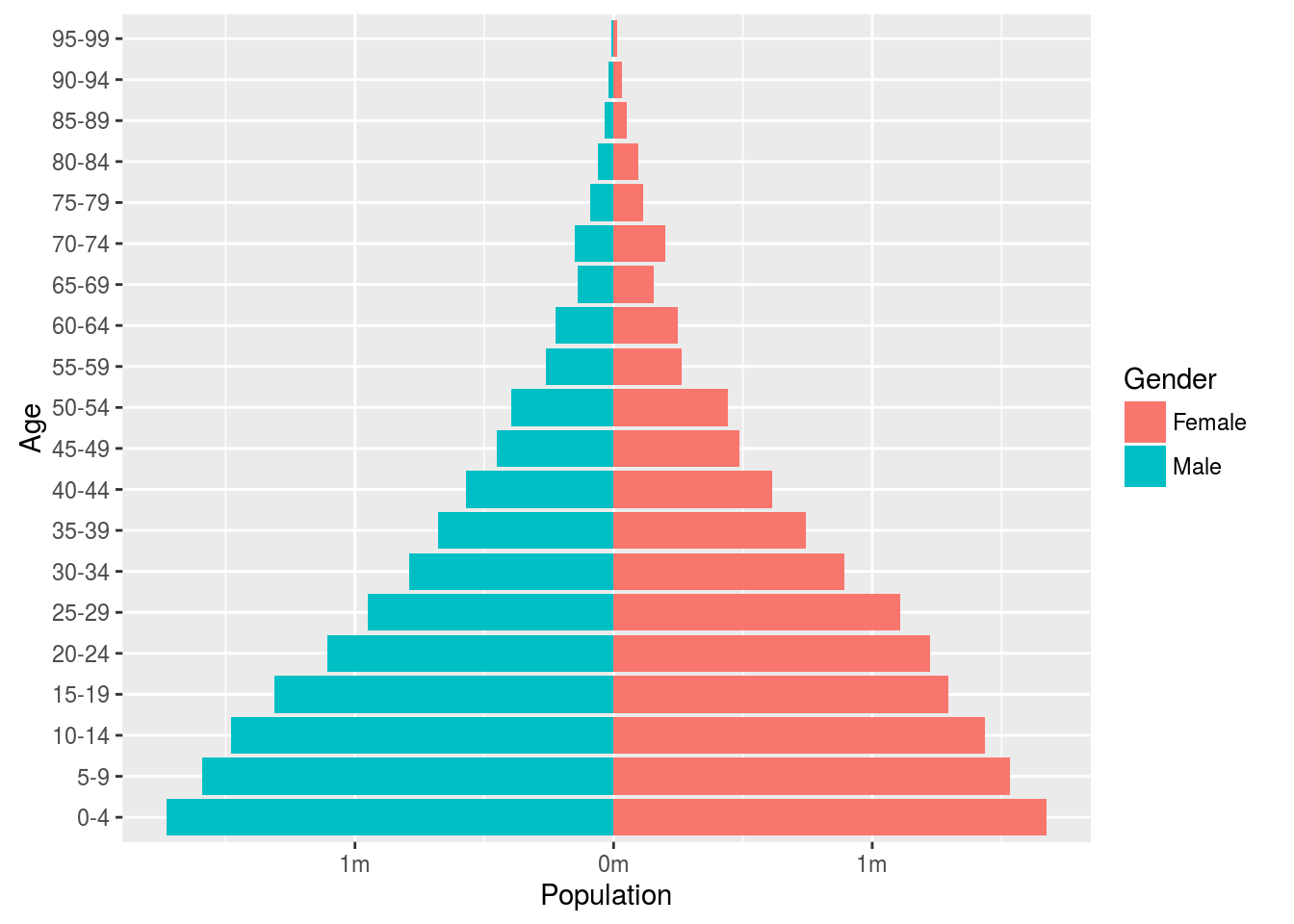## Population pyramides with R

Examples of static and interactive population pyramids using the packages ggplot2 and rCharts and population data from census.gov.

#### 1. ggplot

US Census Bureau’s International Data Base

Start by installing the following packages.

``````install.packages("XML")
install.packages("reshape2")
install.packages("plyr")
install.packages("ggplot2")``````

Then load the installed packages into the active workspace and source some useful functions from my website.

``````library(XML)
library(reshape2)
library(plyr)
library(ggplot2)
source('http://klein.uk/R/Viz/pyramids.R')``````

The following function `getAgeTable` grabs the required population data from the US Census Bureau’s International Data Base and outputs a data frame in the right format for `ggplot2`.

``````popGHcens <- getAgeTable(country = "QA", year = 2015)

pyramidGH <- ggplot(popGHcens, aes(x = Age, y = Population, fill = Gender)) +
geom_bar(data = subset(popGHcens, Gender == "Female"), stat = "identity") +
geom_bar(data = subset(popGHcens, Gender == "Male"), stat = "identity") +
scale_y_continuous(labels = paste0(as.character(c(seq(2, 0, -1), seq(1, 2, 1))), "m")) +
coord_flip()
pyramidGH``````10% sample of 2010 Ghana population census

In an next step, let us try to use the above code snippets to produce the population pyramid for Ghana based on the 2010 census.

``````## load the individual-level age data from the 2010 census

## cut the age variable into age groups with 5-year intervals
popGH\$AGEcut <- cut(popGH\$AGE, breaks = seq(0, 100, 5), right = FALSE)
popGH\$Population <- 10 ## each sampled respondent represents 10 individuals
popGH\$Gender <- popGH\$SEX

## aggregate the data by gender and age group
popGH <- aggregate(formula = Population ~ Gender + AGEcut, data = popGH, FUN = sum)

## sort data by first by gender, then by age groups
popGH <- with(popGH, popGH[order(Gender,AGEcut),])

## for simplicity, add the age group labels we used in popGHcens above
popGH\$Age <- rep(unique(popGHcens\$Age)[1:20], 2)

## only use the three variables age, gender and population from the popGH data
popGH <- popGH[,c("Age","Gender","Population")]

## barplots for male populations goes to the left (thus negative sign)
popGH\$Population <- ifelse(popGH\$Gender == "Male", -1*popGH\$Population, popGH\$Population)

## pyramid charts are two barcharts with axes flipped
pyramidGH2 <- ggplot(popGH, aes(x = Age, y = Population, fill = Gender)) +
geom_bar(data = subset(popGH, Gender == "Female"), stat = "identity") +
geom_bar(data = subset(popGH, Gender == "Male"), stat = "identity") +
scale_y_continuous(labels = paste0(as.character(c(seq(2, 0, -1), seq(1, 2, 1))), "m")) +
coord_flip()
pyramidGH2``````#### 2. rcharts

The package `rCharts` requires that you have Rtools installed in addition to base R. The `rCharts` package is not available on CRAN but can be installed and loaded from GitHub as follows

``````install.packages("devtools")
devtools::install_github("ramnathv/rCharts")
library(rCharts)``````

Plot your pyramid chart by using your country code (e.g. Ghana = `'GH'`) and specifying the colors and years you want to plot.

2.A. Stacked pyramid, by row

``````popGH2 <- getAgeTable2(country = 'IN', year = 2014)
n1 <- nPyramid(dat = popGH2, colors = c('blue', 'silver'))
n1``````

2.B. Normal pyramid, height

``````n2 <- hPyramid(dat = popGH2, colors = c('silver', 'blue'))
n2``````